# Hack 12. Graph Relationships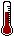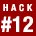Whenever a relationship between two variables is discovered and defined, we can use one variable to guess another. Drawing a regression line allows you to picture the relationship and make predictions.

So, you've just been named assistant regional manager of ice cream sales for 10,000 square feet of prime beachfront retail space along the shores of Sunflower Lake in northeast Kansas. Congratulations! You have a lot of responsibility and many strategic decisions to make about how to maximize profit. One dilemma that you will confront is whether to even open. Being open costs money and uses resources, and if you will sell few ice cream cones that day, it probably won't be worth it to even unlock the service window of your brightly painted plywood shack.

If only there were some way to magically know how good business will be on any given day. As an amateur statistician, you assume there must be a scientific way to guess how many cones will sell without having to actually open for business and test the market for the day. You're in luck. There is a way to make estimates of the value or score on some variable (such as ice cream sales) by using other information.

The key is that the other information must come from a variable that is related to the variable of interest. By drawing a line that shows the relationship among your variables for the days you know, you can look at the line as it extends into the future (or the past) for the days you do not know and guess what will happen. Such a graphic tool is called a regression line.

#### Drawing a Picture of the Future

Observant folks often discover correlations between variables [Hack #11]. The usefulness of knowing that a relationship exists goes beyond descriptive statistics, however.

Imagine that you have data on the activities around Sunflower Lake. Among other things, you have collected information about the amount of ice cream sales under the former assistant regional manager of ice cream sales (in number of ice cream cones sold) and the high temperature for each day (in degrees Fahrenheit). The correlation coefficient that represents the relationship between heat and craving for ice cream should be positive and fairly large. That is, as the heat increases, sales probably increase.

Intuitively, it makes sense that with some experience, you could look at the thermometer and get a sense of how busy the ice cream stand is going to be that day. Once you know that there is a positive or negative relationship between two variables, it makes sense that knowing the score on one will give you a general idea of what the score is on the other.

Once you find a relationship between two variables like this, it is reasonable to assume that the relationship between your two variables is linear. In other words, if you produce a graph with all the possible values of one variable as the X-axis (the horizontal line along the bottom) and all the possible values of the other variable as the Y-axis (the vertical line along the side) and then plot each pair of scores, the resulting dots form an essentially straight line.

#### Connecting the Dots

Figure 2-1 shows a way to graph the relationship between the temperature and ice cream sales at the beach.

##### Figure 2-1. A linear relationship between sales and temperature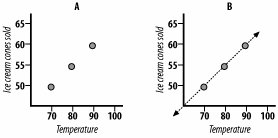Graph A places dots to represent both values on the two variables, based on historic information you have collected. For instance, the lowest dot means that at 70 degrees, 50 ice cream cones were sold. At 90 degrees, 60 cones were sold. There is a clear pattern here, and the relationship looks like a straight line. For every 10-degree jump in temperature, sales go up 5 cones. For every 1-degree change in temperature, there is a 1/2-cone increase in sales. Graph B draws a line based on this rule. The line goes through every dot.

In Figure 2-1, analyze Graph B to get a sense of the power of a regression equation. The line includes territory that is not sampled by the data. For instance, we do not have data for 100-degree days. With the regression equation, though, we can estimate what sales might be. If we place a dot on the line at the 100-degree mark, it appears to match up with the 65-cones mark. Using this regression equation, we could estimate that on 100-degree days, 65 cones would be sold. We could do the same for cooler days. Our graph suggests that on a 60-degree day, 45 cones would be sold.

#### Playing "What If?"

The relationship between heat and cone sales can be expressed mathematically. Our data for graphs A and B in Figure 2-1 look like this:

High temperatureIce cream cones sold
7050
8055
9060

So, let's see how we could build an equation that describes the relationship using numbers. Regression lines are statistical tools, after all. Notice that if we start with 70 degrees, we get 50 cones. If we enter 70 into our formula, we want 50 to be the output. We also want 80 to get us 55, and 90 to get us 60.

I played around with different possibilities using these values in an attempt to figure out what must be done to the input number to get the correct output number. I noticed that the "ice cream cones sold" value was always smaller than the temperature variable, so I wanted an equation that would shrink the temperature. Linear equations require a constant (some value to use in every equation) in order to produce a straight line, so I needed to have a constant in my equation as well. Rather than use trial and error, you could also enter this data into a statistics program, such as SPSS, or a spreadsheet, such as Excel, to produce the correct components. I found that this formula works well:

Cones Sold = 15 + (Temperature x.50)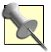Algebraically, if you begin with a constant and then add some standard amount that is altered only through basic mathematical functions, such as multiplication, you will define a straight line that can be graphed.

"What if?" is a fun game to play with regression lines. Enter a value in one end and a guess comes out the other end; you can get an answer even for unrealistic scenarios. Throw some crazy value onto the line, such as 200 degrees, and you can still get an estimate for cone sales: 115!

The regression equation for this relationship would describe a line that could be drawn to show this relationship visually. With real data, the relationship is seldom as clear as it is in our example. (The correlation for our small fictional data set is a perfect 1.0.)In statistics, regression formulas make use of the correlation coefficient, the means, and the standard deviations of both groups of variable scores, regardless of the strength of the relationship in the data set. "Use One Variable to Predict Another" [Hack #13] presents statistical methods for producing a regression equation.

#### Why It Works

The accuracy of these sorts of regression estimates depends on a couple important factors. First, the relationship between variables must be fairly large. Small relationships produce dots all over the place in patterns that aren't straight at all, and a regression line drawn through such a mess misses a lot of dots and is not accurate. Unfortunately, in the social sciences, we don't find very many really strong relationships, so regression predictions tend to produce a certain number of errors. In statistics, errors come with the territory.

Second, the relationship must be at least sort of linear. As in our ice cream cone example, if the nature of the relationship changes somewhere along the regression line, the regression line will miss some of the data. Fortunately, most relationships in the natural world are linear or at least close to it.

#### Where It Doesn't Work

The actual relationship might not be exactly linear, but if it is essentially so, then regression analysis works pretty well. For example, with our ice cream example, maybe there is a certain increase in sales for every degree jump in the temperature. If that increase is the same regardless of where we are on the scale, we'll see a linear relationship. It is possible, though, that sales jump once a certain temperature is reached. Perhaps once it is over 90 degrees at the beach, people really flock to get relief.

Graphs C and D in Figure 2-2 show what happens if the true relationship isn't exactly linear.

##### Figure 2-2. A nonlinear relationship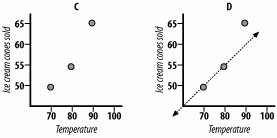Following the requirements of linear regression, the regression equation always produces a straight line and, in this case, two of the dots fall right on it, but one does not. This line does a decent job of explaining the data by picturing the relationship, but because the relationship is not linear, the regression equation makes some errors.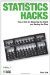Statistics Hacks: Tips & Tools for Measuring the World and Beating the Odds
ISBN: 0596101643
EAN: 2147483647
Year: 2004
Pages: 114
Authors: Bruce Frey

Similar book on Amazon